# Balbharati solutions for Mathematics 2 Geometry 10th Standard SSC Maharashtra State Board chapter 1 - Similarity [Latest edition]

#### Chapters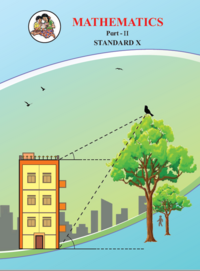## Chapter 1: Similarity

Practice Set 1.1Practice Set 1.2Practice Set 1.3Practice Set 1.4Problem Set 1
Practice Set 1.1 [Pages 5 - 6]

### Balbharati solutions for Mathematics 2 Geometry 10th Standard SSC Maharashtra State Board Chapter 1 Similarity Practice Set 1.1 [Pages 5 - 6]

Practice Set 1.1 | Q 1 | Page 5

Base of a triangle is 9 and height is 5. Base of another triangle is 10 and height is 6. Find the ratio of areas of these triangles.

Practice Set 1.1 | Q 2 | Page 6

In the given figure, BC ⊥ AB, AD ⊥ AB, BC = 4, AD = 8, then find $\frac{A\left( ∆ ABC \right)}{A\left( ∆ ADB \right)} .$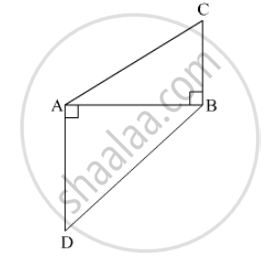Practice Set 1.1 | Q 3 | Page 6

In adjoining figure, seg PS ⊥ seg RQ seg QT ⊥ seg PR. If RQ = 6, PS = 6 and PR = 12, then Find QT.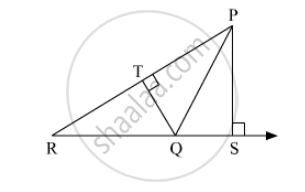Practice Set 1.1 | Q 4 | Page 6

In the following figure, AP ⊥ BC, AD || BC, then Find A(∆ABC): A(∆BCD).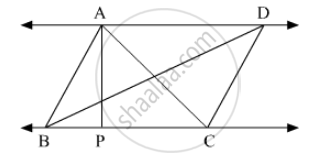Practice Set 1.1 | Q 5 | Page 6

In adjoining figure PQ ⊥ BC, AD⊥ BC then find following ratios.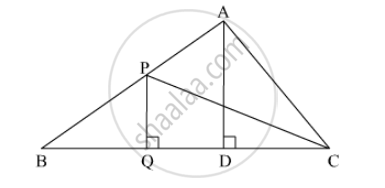(i) $\frac{A\left( ∆ PQB \right)}{A\left( ∆ PBC \right)}$

(ii) $\frac{A\left( ∆ PBC \right)}{A\left( ∆ ABC \right)}$

(iii) $\frac{A\left( ∆ ABC \right)}{A\left( ∆ ADC \right)}$

(iv) $\frac{A\left( ∆ ADC \right)}{A\left( ∆ PQC \right)}$

Practice Set 1.2 [Pages 13 - 15]

### Balbharati solutions for Mathematics 2 Geometry 10th Standard SSC Maharashtra State Board Chapter 1 Similarity Practice Set 1.2 [Pages 13 - 15]

Practice Set 1.2 | Q 1.1 | Page 13

Identify ray PM is the bisector of ∠QPR.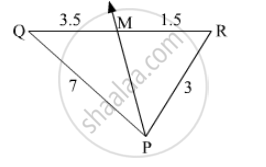Practice Set 1.2 | Q 1.2 | Page 13

Identify ray PM is the bisector of ∠QPR.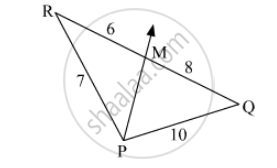Practice Set 1.2 | Q 1.3 | Page 13

Identify ray PM is the bisector of ∠QPR.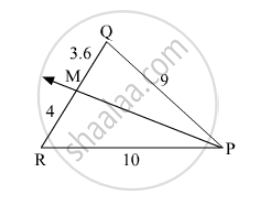Practice Set 1.2 | Q 2 | Page 13

In ∆PQR, PM = 15, PQ = 25 PR = 20, NR = 8. State whether line NM is parallel to side RQ. Give reason.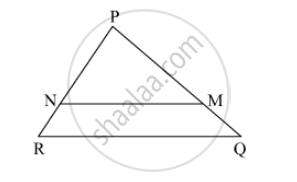Practice Set 1.2 | Q 3 | Page 14

In ∆MNP, NQ is a bisector of ∠N. If MN = 5, PN = 7 MQ = 2.5 then Find QP.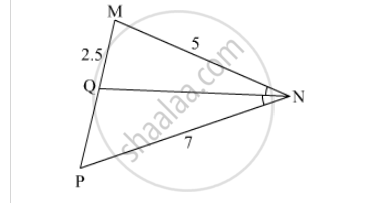Practice Set 1.2 | Q 4 | Page 14

Measures of some angles in the figure are given. Prove that $\frac{AP}{PB} = \frac{AQ}{QC}$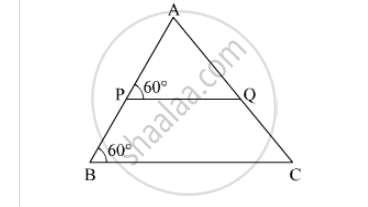Practice Set 1.2 | Q 5 | Page 14

In trapezium ABCD, side AB || side PQ || side ∆C, AP = 15, PD = 12, QC = 14, Find BQ.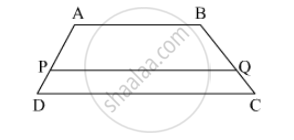Practice Set 1.2 | Q 6 | Page 14

Find QP using given information in the figure.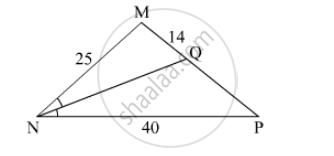Practice Set 1.2 | Q 7 | Page 14

In the given figure, if AB || CD || FE then Find x and AE.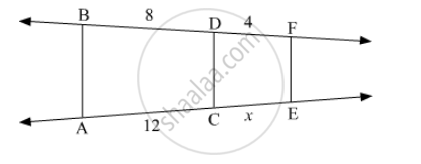Practice Set 1.2 | Q 8 | Page 15

In ∆LMN, ray MT bisects ∠LMN If LM = 6, MN = 10, TN = 8, then Find LT.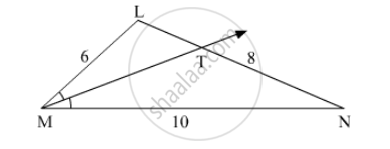Practice Set 1.2 | Q 9 | Page 15

In ∆ABC, seg BD bisects ∠ABC. If AB = x, BC = + 5, AD = – 2, DC = + 2, then find the value of x.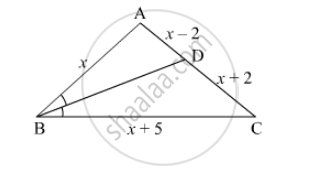Practice Set 1.2 | Q 10 | Page 15

In the given figure, X is any point in the interior of triangle. Point X is joined to vertices of triangle. Seg PQ || seg DE, seg QR || seg EF. Fill in the blanks to prove that, seg PR || seg DF.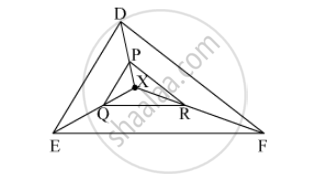Proof :  In Δ XDE, PQ || DE         ........ ___________

therefore "XP"/([    ]) = ([    ])/"QE"  ..... (I) (Basic proportionality theorem)

In Δ XEE, QR || EF                    ........  _________

therefore ([     ])/([     ]) = ([      ])/([      ])    .......(II) _________________

therefore ([     ])/([     ]) = ([      ])/([      ])   ....... from (I) and  (II)

∴ seg PR || seg DE           ........... (converse of basic proportionality theorem)

Practice Set 1.2 | Q 11 | Page 15

In ∆ABC, ray BD bisects ∠ABC and ray CE bisects ∠ACB. If seg AB ≅ seg AC then prove that ED || BC.

Practice Set 1.3 [Pages 21 - 22]

### Balbharati solutions for Mathematics 2 Geometry 10th Standard SSC Maharashtra State Board Chapter 1 Similarity Practice Set 1.3 [Pages 21 - 22]

Practice Set 1.3 | Q 1 | Page 21

In the given figure, ∠ABC = 75°, ∠EDC = 75° state which two triangles are similar and by which test? Also write the similarity of these two triangles by a proper one to one correspondence.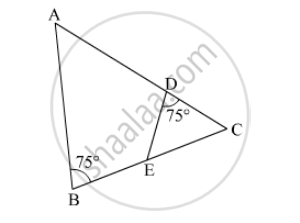Practice Set 1.3 | Q 2 | Page 21

Are the triangles in the given figure similar? If yes, by which test ?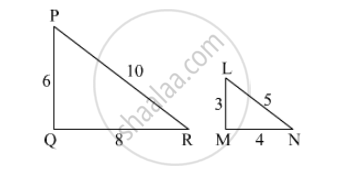Practice Set 1.3 | Q 3 | Page 21

As shown in the given figure, two poles of height 8 m and 4 m are perpendicular to the ground. If the length of shadow of smaller pole due to sunlight is 6 m then how long will be the shadow of the bigger pole at the same time ?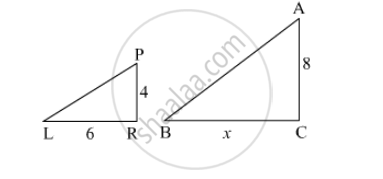Practice Set 1.3 | Q 4 | Page 21

In ∆ABC, AP ⊥ BC, BQ ⊥ AC B– P–C, A–Q – C then prove that, ∆CPA ~ ∆CQB. If AP = 7, BQ = 8, BC = 12 then Find AC.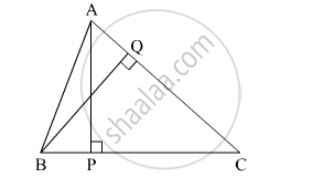Practice Set 1.3 | Q 5 | Page 22

In trapezium PQRS, side PQ || side SR, AR = 5AP, AS = 5AQ then prove that, SR = 5PQ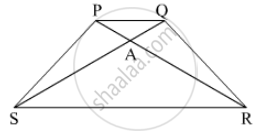Practice Set 1.3 | Q 6 | Page 22

In trapezium ABCD, side AB || side DC, diagonals AC and BD intersect in point O. If AB = 20, DC = 6, OB = 15 then Find OD.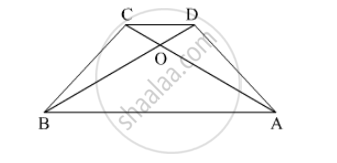Practice Set 1.3 | Q 7 | Page 22

◻ABCD is a parallelogram point E is on side BC. Line DE intersects ray AB in point T. Prove that DE × BE = CE × TE.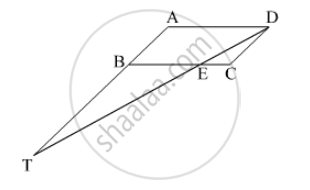Practice Set 1.3 | Q 8 | Page 22

In the given figure, seg AC and seg BD intersect each other in point P and $\frac{AP}{CP} = \frac{BP}{DP}$ Prove that, ∆ABP ~ ∆CDP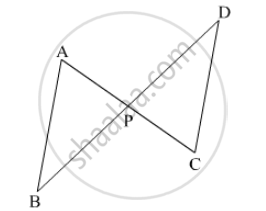Practice Set 1.3 | Q 9 | Page 22

In the given figure, in ∆ABC, point D on side BC is such that, ∠BAC = ∠ADC. Prove that, CA2 = CB × CD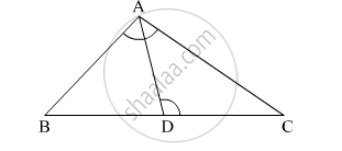Practice Set 1.4 [Page 25]

### Balbharati solutions for Mathematics 2 Geometry 10th Standard SSC Maharashtra State Board Chapter 1 Similarity Practice Set 1.4 [Page 25]

Practice Set 1.4 | Q 1 | Page 25

The ratio of corresponding sides of similar triangles is 3 : 5; then Find the ratio of their areas.

Practice Set 1.4 | Q 2 | Page 25

If ∆ABC ~ ∆PQR and AB : PQ = 2 : 3, then fill in the blanks.

$\frac{A\left( ∆ ABC \right)}{A\left( ∆ PQR \right)} = \frac{{AB}^2}{......} = \frac{2^2}{3^2} = \frac{......}{.......}$

Practice Set 1.4 | Q 3 | Page 25

If ∆ABC ~ ∆PQR, A (∆ABC) = 80, A (∆PQR) = 125, then fill in the blanks. $\frac{A\left( ∆ ABC \right)}{A\left( ∆ . . . . \right)} = \frac{80}{125} \therefore \frac{AB}{PQ} = \frac{......}{......}$

Practice Set 1.4 | Q 4 | Page 25

∆LMN ~ ∆PQR, 9 × A (∆PQR ) = 16 × A (∆LMN). If QR = 20 then Find MN.

Practice Set 1.4 | Q 5 | Page 25

Areas of two similar triangles are 225 sq.cm. 81 sq.cm. If a side of the smaller triangle is 12 cm, then Find corresponding side of the bigger triangle.

Practice Set 1.4 | Q 6 | Page 25

∆ABC and ∆DEF are equilateral triangles. If A(∆ABC) : A (∆DEF) = 1 : 2 and AB = 4, find DE.

Practice Set 1.4 | Q 7 | Page 25

In the given figure 1.66, seg PQ || seg DE, A(∆PQF) = 20 units, PF = 2 DP, then Find A(◻DPQE) by completing the following activity.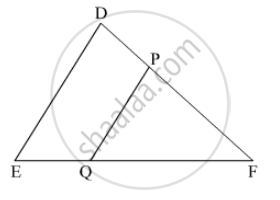Problem Set 1 [Pages 26 - 29]

### Balbharati solutions for Mathematics 2 Geometry 10th Standard SSC Maharashtra State Board Chapter 1 Similarity Problem Set 1 [Pages 26 - 29]

Problem Set 1 | Q 1.1 | Page 26

Select the appropriate alternative.
In ∆ABC and ∆PQR, in a one to one correspondence $\frac{AB}{QR} = \frac{BC}{PR} = \frac{CA}{PQ}$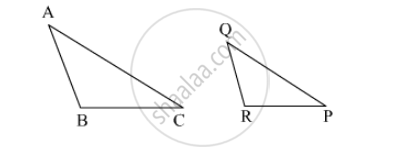• ∆PQR ~ ∆ABC

• ∆PQR ~ ∆CAB

• ∆CBA ~ ∆PQR

• ∆BCA ~ ∆PQR

Problem Set 1 | Q 1.2 | Page 26

If in ∆DEF and ∆PQR, ∠D ≅ ∠Q, ∠R ≅ ∠E then which of the following statements is false?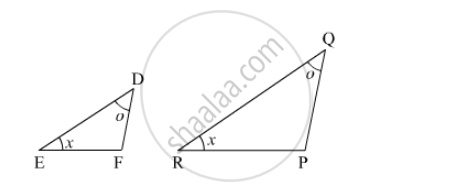• $\frac{EF}{PR} = \frac{DF}{PQ}$

• $\frac{DE}{PQ} = \frac{EF}{RP}$

• $\frac{DE}{QR} = \frac{DF}{PQ}$

• $\frac{EF}{RP} = \frac{DE}{QR}$

Problem Set 1 | Q 1.3 | Page 26

In ∆ABC and ∆DEF ∠B = ∠E, ∠F = ∠C and AB = 3DE then which of the statements regarding the two triangles is true ?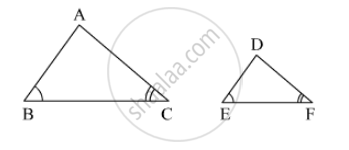• The triangles are not congruent and not similar

• The triangles are similar but not congruent.

• The triangles are congruent and similar.

• None of the statements above is true.

Problem Set 1 | Q 1.4 | Page 26

∆ABC and ∆DEF are equilateral triangles, A (∆ABC) : A (∆DEF) = 1 : 2
If AB = 4 then what is length of DE?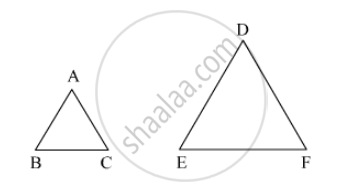• $2\sqrt{2}$

• $4\sqrt{2}$

Problem Set 1 | Q 1.5 | Page 26

In the given figure, seg XY || seg BC, then which of the following statements is true?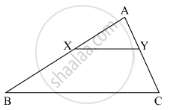• "AB"/"AC" = "AX"/"AY"

• "AX"/"XB" ="AY"/"AC"

• "AX"/"YC" = "AY"/"XB"

• "AB"/"YC" = "AC"/"XB"

Problem Set 1 | Q 2.1 | Page 27

In ∆ABC, B – D – C and BD = 7, BC = 20 then Find following ratio.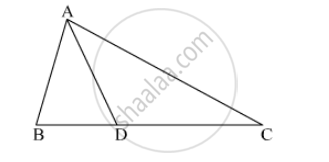$\frac{A\left( ∆ ABD \right)}{A\left( ∆ ADC \right)}$

Problem Set 1 | Q 2.2 | Page 27

In ∆ABC, B – D – C and BD = 7, BC = 20 then Find following ratio.$\frac{A\left( ∆ ABD \right)}{A\left( ∆ ABC \right)}$

Problem Set 1 | Q 2.3 | Page 27

In ∆ABC, B – D – C and BD = 7, BC = 20 then Find following ratio.$\frac{A\left( ∆ ADC \right)}{A\left( ∆ ABC \right)}$

Problem Set 1 | Q 3 | Page 27

Ratio of areas of two triangles with equal heights is 2 : 3. If base of the smaller triangle is 6 cm then what is the corresponding base of the bigger triangle ?

Problem Set 1 | Q 4 | Page 27

In the given figure, ∠ABC = ∠DCB = 90° AB = 6, DC = 8 then $\frac{A \left( ∆ ABC \right)}{A \left( ∆ DCB \right)} = ?$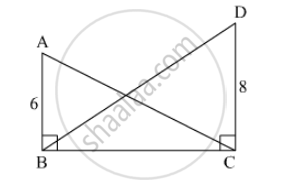Problem Set 1 | Q 5 | Page 27

In the given figure, PM = 10 cm A(∆PQS) = 100 sq.cm A(∆QRS) = 110 sq.cm then Find NR.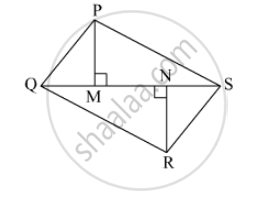Problem Set 1 | Q 6 | Page 27

∆MNT ~ ∆QRS. Length of altitude drawn from point T is 5 and length of altitude drawn from point S is 9. Find the ratio $\frac{A\left( ∆ MNT \right)}{A\left( ∆ QRS \right)}$

Problem Set 1 | Q 7 | Page 28

In the given figure, A – D– C and B – E – C seg DE || side AB If AD = 5, DC = 3, BC = 6.4 then Find BE.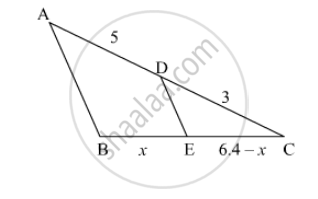Problem Set 1 | Q 8 | Page 28

In the given figure, seg PA, seg QB, seg RC and seg SD are perpendicular to line AD. AB = 60, BC = 70, CD = 80, PS = 280 then Find PQ, QR and RS.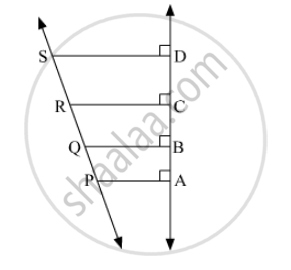Problem Set 1 | Q 9 | Page 28

In ∆PQR seg PM is a median. Angle bisectors of ∠PMQ and ∠PMR intersect side PQ and side PR in points X and Y respectively. Prove that XY || QR.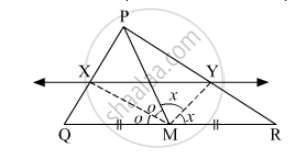Problem Set 1 | Q 10 | Page 29

In the given fig, bisectors of ∠B and ∠C of ∆ABC intersect each other in point X. Line AX intersects side BC in point Y. AB = 5, AC = 4, BC = 6 then find $\frac{AX}{XY}$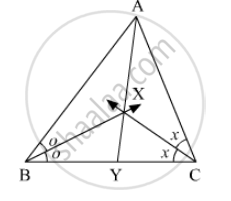Problem Set 1 | Q 11 | Page 29

In ▢ABCD, seg AD || seg BC. Diagonal AC and diagonal BD intersect each other in point P. Then show that $\frac{AP}{PD} = \frac{PC}{BP}$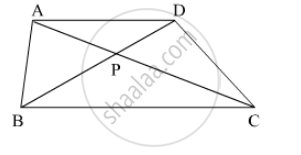Problem Set 1 | Q 12 | Page 29

In the given fig, XY || seg AC. If 2AX = 3BX and XY = 9. Complete the activity to Find the value of AC.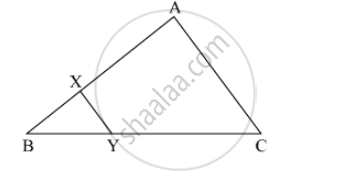Problem Set 1 | Q 13 | Page 29

In the given figure, the vertices of square DEFG are on the sides of ∆ABC. ∠A = 90°. Then prove that DE2 = BD × EC (Hint: Show that ∆GBD is similar to ∆CFE. Use GD = FE = DE.)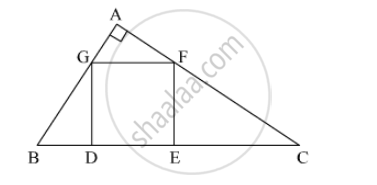## Chapter 1: Similarity

Practice Set 1.1Practice Set 1.2Practice Set 1.3Practice Set 1.4Problem Set 1## Balbharati solutions for Mathematics 2 Geometry 10th Standard SSC Maharashtra State Board chapter 1 - Similarity

Balbharati solutions for Mathematics 2 Geometry 10th Standard SSC Maharashtra State Board chapter 1 (Similarity) include all questions with solution and detail explanation. This will clear students doubts about any question and improve application skills while preparing for board exams. The detailed, step-by-step solutions will help you understand the concepts better and clear your confusions, if any. Shaalaa.com has the Maharashtra State Board Mathematics 2 Geometry 10th Standard SSC Maharashtra State Board solutions in a manner that help students grasp basic concepts better and faster.

Further, we at Shaalaa.com provide such solutions so that students can prepare for written exams. Balbharati textbook solutions can be a core help for self-study and acts as a perfect self-help guidance for students.

Concepts covered in Mathematics 2 Geometry 10th Standard SSC Maharashtra State Board chapter 1 Similarity are Properties of Ratios of Areas of Two Triangles, Similarity of Triangles, Similar Triangles, Similarity Triangle Theorem, Areas of Two Similar Triangles, Property of three parallel lines and their transversals, Property of an Angle Bisector of a Triangle, Basic Proportionality Theorem Or Thales Theorem, Converse of Basic Proportionality Theorem, Appolonius Theorem, Application of Pythagoras Theorem in Acute Angle and Obtuse Angle, Areas of Similar Triangles, Similarity.

Using Balbharati 10th Standard Board Exam solutions Similarity exercise by students are an easy way to prepare for the exams, as they involve solutions arranged chapter-wise also page wise. The questions involved in Balbharati Solutions are important questions that can be asked in the final exam. Maximum students of Maharashtra State Board 10th Standard Board Exam prefer Balbharati Textbook Solutions to score more in exam.

Get the free view of chapter 1 Similarity 10th Standard Board Exam extra questions for Mathematics 2 Geometry 10th Standard SSC Maharashtra State Board and can use Shaalaa.com to keep it handy for your exam preparation